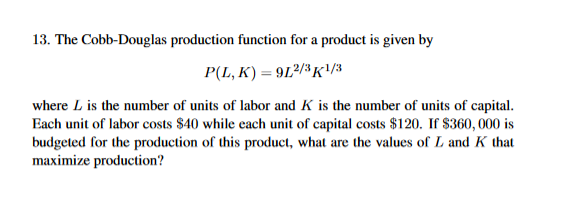# 13. The Cobb-Douglas production function for a product is given byP(L, K) 912/K1where L is the number of units of labor and K is the number of units of capital.Each unit of labor costs S40 while each unit of capital costs \$120. If S360, 000 isbudgeted for the production of this product, what are the values of L and K thatmaximize production?

Question
98 viewshelp_outlineImage Transcriptionclose13. The Cobb-Douglas production function for a product is given by P(L, K) 912/K1 where L is the number of units of labor and K is the number of units of capital. Each unit of labor costs S40 while each unit of capital costs \$120. If S360, 000 is budgeted for the production of this product, what are the values of L and K that maximize production? fullscreen
check_circle

Step 1

To find the values of L and K that maximize production if S360, 000 is budgeted for the production of this product. Here L is the number of units of labor and K is the number of units of capital. Each unit of labor costs S40 while each unit of capital costs \$120.Also the  production function for a product is given by P(L, K). The total budject can be mathematically expressed as follows:

Step 2

Use the Lagrange multipliers subjec t to the constraint g(L,K) = 40L+120K=360000. Also equate PLλgL and  PKλgK.

Step 3

Simplify again to obtain the values of&nb...

### Want to see the full answer?

See Solution

#### Want to see this answer and more?

Solutions are written by subject experts who are available 24/7. Questions are typically answered within 1 hour.*

See Solution
*Response times may vary by subject and question.
Tagged in

### Algebra